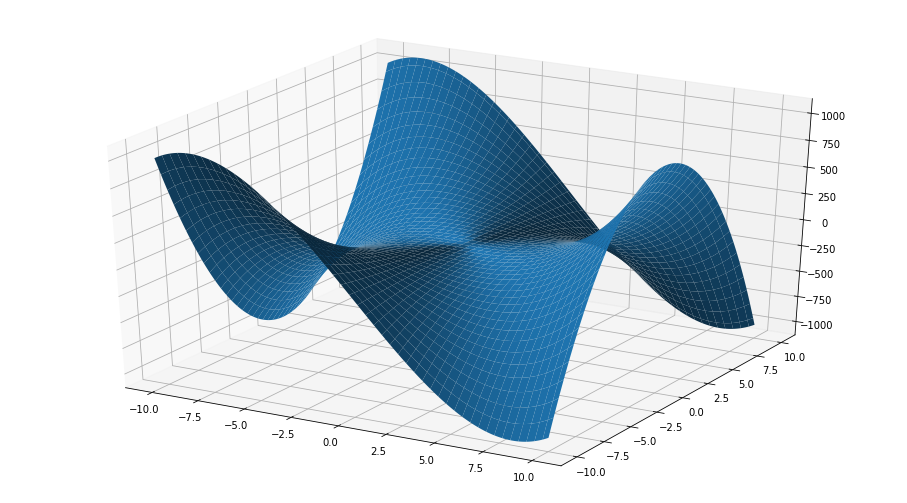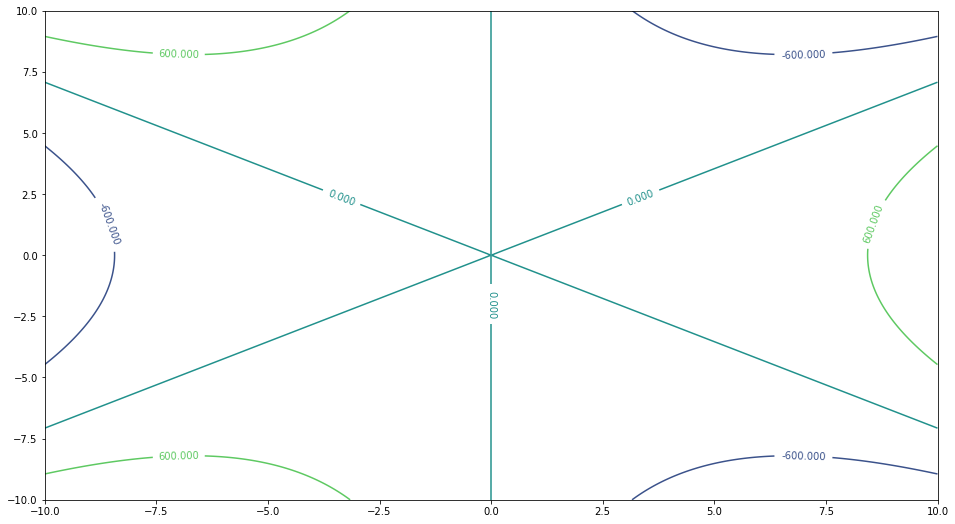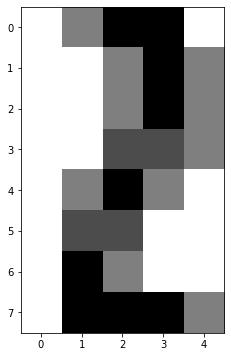# 03.02 Plots in Three Dimensions¶

Working with three dimensional plotting can be confusing at best. We only have a projection into two dimensions at any single time, and the projection may not be a good representation. That said, three dimensional plots are useful in certain circumstances and we shall have some exposure to them.plt-dots-3d.svg

In matplotlib the three dimensional plotting was a later addition, hence the imports work in a slightly different manner. The import of the mplot3d toolkit for three dimensional plotting alters the structure of matplotlib figures and allows for three dimensional axes to be added to the figure.

In :
import numpy as np
import matplotlib.pyplot as plt
%matplotlib inline
from mpl_toolkits import mplot3d


Let us get our hands dirty and plot a classic three dimensional function, the monkey saddle:

$$z = x^3 - 2xy^2$$

Note that there exist a value for $z$ for ever permutation of values of $x$ and $y$. In other words, $z$ is a surface projected from every point on the $xy$-plane. In order to achieve this we need np.meshgrid, which creates a permutation of every point in $x$ and $y$ and returns the full set of permutations. The plotting itself happens by using the matplotlib figure as with every other plot. The difference is that we explicitly add a set of axes with the 3d projection. On these extended axes we can then performs three dimensional plotting with specific functions, here a surface plot.

In :
x = np.linspace(-10, 10, 1024)
y = np.linspace(-10, 10, 512)
gx, gy = np.meshgrid(x, y)

z = gx**3 - 2*gx*gy**2

fig = plt.figure(figsize=(16, 9))
ax = fig.add_subplot(111, projection='3d')
ax.plot_surface(gx, gy, z)
gx.shape, gy.shape, z.shape

Out:
((512, 1024), (512, 1024), (512, 1024))The function is called a monkey saddle because it has extra space for the tail of the monkey sitting in it.

Okay, we can plot that mesh grid but what does np.meshgrid actually does? We shall attempt a simpler example, here the surface function is trivial.

$$z = 3x + y$$
In :
x = np.array([1, 2, 3])
y = np.array([7, 9])
gx, gy = np.meshgrid(x, y)
z = 3*gx + gy
gx, gy, z

Out:
(array([[1, 2, 3],
[1, 2, 3]]),
array([[7, 7, 7],
[9, 9, 9]]),
array([[10, 13, 16],
[12, 15, 18]]))

One can see that the mesh grid generated all permutations between $1, 2, 3$ and $7, 9$. Then the function is evaluated on the $2 x 3$ matrix. Note that there is no need for the number of values in $x$ and in $y$ to be the same, the permutations will always result in matrices of shape valued as the number of values in $x$ and the number of values in $y$.

The evaluation of the function itself is easier to see if we reshape the permutations. Here we see columns for $x$, $y$ and the evaluated $z$.

In :
np.c_[gx.reshape(-1), gy.reshape(-1), z.reshape(-1)]

Out:
array([[ 1,  7, 10],
[ 2,  7, 13],
[ 3,  7, 16],
[ 1,  9, 12],
[ 2,  9, 15],
[ 3,  9, 18]])

The mesh grid in NumPy is not limited to three dimensions. As many things in NumPy, the limit is on human imagination on what higher dimensional mesh grids represent rather than the tool. That said a use for a more than three dimensional mesh grid is a vector field, and we will see one soon.

First we need to notice that a surface plot is not the only way in which one may plot a three dimensional function such a the monkey saddle. We can attempt a plot of contour curves.

Here we use normal matplotlib plotting and use the contour function to build the contour lines. The level= argument gives the number of regions between the lines.

In :
x = np.linspace(-10, 10, 1024)
y = np.linspace(-10, 10, 512)
gx, gy = np.meshgrid(x, y)

z = gx**3 - 2*gx*gy**2

label_locations = [
( 7., -8.),
(-9.,  1.),
( 7.,  8.),
( 0., -2.),
(-3.,  4.),
( 3.,  4.),
(-7., -8.),
( 9.,  1.),
(-7.,  8.),
]
fig, ax = plt.subplots(figsize=(16, 9))
c = ax.contour(gx, gy, z, levels=3)
cl = ax.clabel(c, manual=label_locations)
gx.shape, gy.shape, z.shape

Out:
((512, 1024), (512, 1024), (512, 1024))A recurring pattern in matplotlib is the use of the return value from the plotting function to modify its appearance. Here we use clabel to label the contours. That said, the clunkiness of matplotlib shows here. The clabel function has the bad habit of placing labels in overlapping locations or even in location out of the graph. Above we manually force the locations of all labels.

The contour graph for the monkey saddle shows the three way saddle: three contour lines at $600$ and three contour lines at $-600$. Yet, the surface plot for this specific function makes for a better visualization. The monkey saddle is the kind of function badly represented by its contour lines.

Another set of three dimensional plots are images. What? Yes, images, good flat images. In an image we have the two coordinates of each pixel and a third dimension as the value of that pixel. Sometimes, plotting data as an image or using an image as data itself in such a three dimensional view is a viable approach.

Let's have a look at a simple array containing some values between $0$ and $1$.

In :
x = np.array([
[0., .5,  1., 1., 0.],
[0., 0.,  .5, 1., .5],
[0., 0.,  .5, 1., .5],
[0., 0.,  .7, .7, .5],
[0., .5,  1., .5, 0.],
[0., .7,  .7, 0., 0.],
[0., 1.,  .5, 0., 0.],
[0., 1.,  1., 1., .5],
])
fig, ax = plt.subplots(figsize=(14, 6))
ax.imshow(x, cmap='binary');And the use of imshow gives us a reasonable description of a number $2$ in a $4$ by $8$ pixel display. But wait, that was a matrix of values, and a matrix ought to have two dimensions! To be fair, the dimensionality of tabular data, e.g. a matrix, depends on the interpretation of the data itself. What we care about right now is the number of dimensions presented in the displayed graph, and in the graph of the number $2$ above that number of dimensions is $3$: horizontal position of pixel, vertical position of pixel, and value of pixel.

We will eventually build a better description of dimensionality. But for now, we will just argue that NumPy dimensions are the number of values in the shape of the array, and that the plot dimensions are the number of distinct values we can read about a single point on the graph itself. And for now we will not link one dimensionality to the other.

We will also come back to the cmap= argument soon enough. For the time being we will just argue that binary here means black and white. And we need to use it because the default value for cmap= make a colorful image.

To round this dimensionality trip let us look at a six dimensional example: To every point in three dimensions we will assign a three dimensional vector. Wherever you are on the function below, if you follow the vectors you will reach zero but you will not necessarily go through the shortest path.

The vector field below tend to move towards the $x$ and the $y$ axes, i.e. one moves toward the $x$ or $y$ axis with only negligible change in the $z$ coordinates. Once a point has reached one of the axes ($x=0$, $y=0$, or both) further vectors point towards the direction of $z=0$. If represented by surfaces, the function would be an infinite number of concentric curved funnels towards the origin.

Below we use the scatter to plot the points in three dimensions, here the olive dots. Then we use quiver (as in a bag of arrows) to plot all vectors. quiver receives six coordinate arguments: three positions for the tail of the vector and then three more values for the value of the vector in each direction. To allow for better visualization we normalize the vector values between $0$ and $1$ with normalize=True; and set camera elevation (on the $z$ axis) and azimuth (on the $xy$-plane) with view_init.

In :
x, y, z = np.meshgrid(
np.linspace(-5, 5, 20),
np.linspace(-5, 5, 20),
np.array([1., 2., 3.]))
u = (10 - 1/x - 1/y)*(-x)
v = (10 - 1/x - 1/y)*(-y)
w = 2*(1/np.abs(x) + 1/np.abs(y))*(2 - z)

fig = plt.figure(figsize=(20, 12))
ax = fig.add_subplot(111, projection='3d')
ax.scatter(x, y, z, color='olive')
ax.quiver(x, y, z, u, v, w, length=0.2, normalize=True, color='seagreen')
ax.view_init(elev=20., azim=30)
x.shape, y.shape, z.shape, u.shape, v.shape, w.shape

Out:
((20, 20, 3), (20, 20, 3), (20, 20, 3), (20, 20, 3), (20, 20, 3), (20, 20, 3))